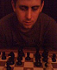Math Is Fun Forum

Discussion about math, puzzles, games and fun.   Useful symbols: ÷ × ½ √ ∞ ≠ ≤ ≥ ≈ ⇒ ± ∈ Δ θ ∴ ∑ ∫  π  -¹ ² ³ °

You are not logged in.

#1 2011-06-14 09:11:43

studymaths
MemberRegistered: 2010-04-11
Posts: 32

Math one-liners

I'm trying to gather as many one - liner maths jokes together as I can so I thought I'd share the ones I have collected so far...apologies to the javascript layout!

joke="Why was 6 afraid of 7?";
joke="What do you call a boiling kettle on top of a mountain?";
joke="There a 10 types of people.";
joke="BREAKING NEWS!";
joke="Have you heard the joke about the statistician?";
joke="What happens when you take the circumference of your Halloween lantern and divide it by its diameter?";
joke="What do mermaid mathematicians wear?";
joke="Why did the chicken cross the Mobius strip?";
joke="There were 99 people on a boat when it capsized. How many were left on the boat?";
joke="What does a constipated mathematician do?";
joke="What did the zero say to the eight?";
joke="Pi says: 'Get real.'";
joke="Why was the maths book sad?";
joke="An infinite crowd of mathematicians enter a pub. The first one orders a pint, the second one a half pint, the third one a quarter pint and so on.";
joke="Why don't you find many mathematicians on the beach?";
joke="Why did the pupil eat her homework?";
joke="What does a clock do when it's hungry?";
joke="Why is two the oddest prime?";
joke="Teacher: 'Along the corridor up the stairs'";
joke="A statistician had his head in an oven and his feet in the freezer.";
joke="What's the king of the pencil case?";
joke="What's a sheeps favourite graph?";
joke="What did the obtuse angle say to the smaller angle?";
joke="What T.V. programme do statisticians watch?"
joke="Why was the prism angry?";
joke="How much is the cheapest carpet at the metric store?";
joke="How do geometry teachers travel?";
joke="What is a mathematians favourite food?";
joke="What do you call a leg that is perpendicular to a foot?";
joke="What did one maths book say to the other?";
joke="What state has the most maths teachers?";
joke="Did you hear the joke about the infinite line?";
joke="Why did the two vectors start an internet-based company?";
joke="Why did the integer get mad at his wife?";
joke="Shocking statistic...";
joke="F(x)= 2X+3 walks into a bar and orders a round of drinks and asks for some sanwiches.";
joke="Who invented King Arthur's round table?";
joke="How do you tell that you are in the hands of the Mathematical Mafia?";
joke="How many numerical analysts does it take to change a lightbulb?";
joke="What's the difference between Robbie Williams and a maths teacher?";
joke="Where are mathematicians buried?";
joke="Decimals have a point.";
joke="Why don't they serve beer at mathematicians' parties?";

And now all the punch lines...

punch="Because 7, 8, 9!";
punch="A high-pot-in-use!";
punch="Those that understand binary and those that don't.";
punch="A new prime number has been discovered three times larger than the previous record.";
punch="You get Pumpkin Pie.";
punch="Algebras.";
punch="To get to the same side.";
punch="66.";
punch="Work it out with a pencil.";
punch="Nice belt.";
punch="Because it had too many problems.";
punch="The bar man says 'I get ya' and pours two pints.";
punch="Because they can get a tan with just a sine and cosine.";
punch="Because the teacher said it was a piece of cake.";
punch="It goes back four seconds.";
punch="It's the only one that's even!";
punch="Pupil: 'Sir, I live in a bungalow'";
punch="On average he felt fine.";
punch="The ruler.";
punch="A baaaa chart.";
punch="Correlation street.";
punch="One cent a metre.";
punch="In a plane.";
punch="Pi."
punch="A right ankle.";
punch="Man I have a lot of problems.";
punch="Mathachussets.";
punch="It doesn't have a point.";
punch="Call 1-800-[(10x)(13i)^2]-[sin(xy)/2.362x].";
punch="They thought they had a good dot product.";
punch="Because she was being irrational.";
punch="50% of schools are below average.";
punch="It's got square roots.";
punch="The barman replies sorry we don't cater for functions.";
punch="Sir Cumference.";
punch="They make you an offer that you can't understand.";
punch="0.99987 after four iterations.";
punch="The Symmetry.";
punch="Calculus has it's limits.";
punch="Because it's not safe to drink and derive.";
punch="They're the first sine of madness."

Any more for any more?

Offline

#2 2011-06-14 14:52:43

soroban
Member
Registered: 2007-03-09
Posts: 452

.

. .

.

Offline

#3 2011-07-14 16:22:53

soroban
Member
Registered: 2007-03-09
Posts: 452

.

.

Offline